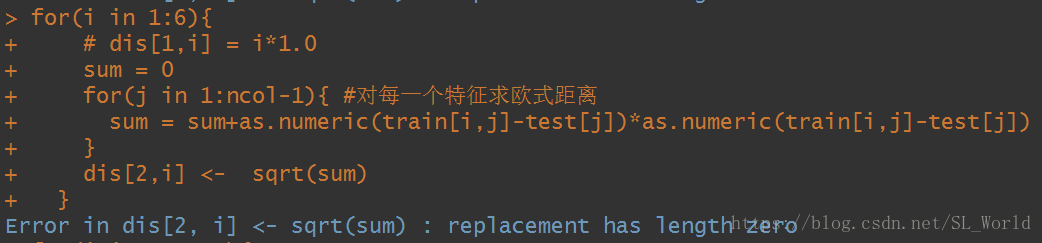# Analysis of R language error replacement has length zero problem

I encountered this problem before when I was doing a for loop, where the second layer of the loop is `, ncol=3`, so here the loop is going to be `for(j in 1:2)` and then the assignment matrix is the following error.

After, it was found on the Internet that the error was caused by improper alignment of array or matrix or out-of-bounds subscripts. After checking the code, it was found that there was indeed a small error, that is, `for(j in 1:ncol-1)` is equivalent to `for(j in 0:1)`. Obviously, an error will be reported when assigning the value. `for(j in 1: ncol-1))` correct.